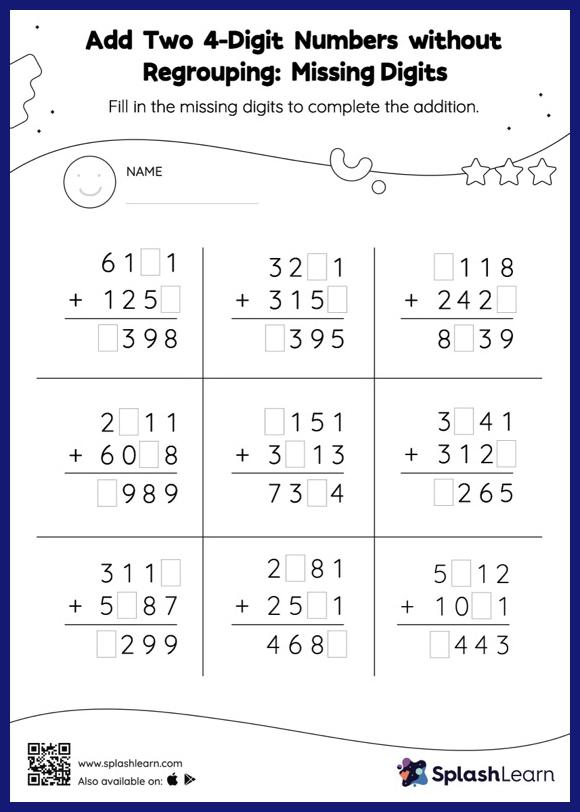# Add Two 4-Digit Numbers without Regrouping: Missing Digits Worksheet

Home > Add Two 4-Digit Numbers without Regrouping: Missing DigitsThis worksheet gives students an opportunity to apply the concepts of addition to add two 4-digit numbers without regrouping. To find the missing number on this worksheet, students can utilize the count-on strategy or the connection between addition and subtraction. In add two 4-digit numbers without regrouping worksheet, they do not need to regroup numbers to reach the solution. This worksheet is about practicing the column method in which numbers are written one on top of another. In this method, students use their place value understanding to solve the problems.